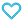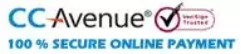# Rank Up Physics JEE Main & Advanced Waves and Thermodynamics

300.00

• Paper Book

Discount offer on this book in a bundle, click to view

MTG’s Rank Up Physics for JEE Main & Advanced is a Physics book series comprising 5 books. The series is created by Physics experts to ensure that a JEE (M+A) aspirant can cover the Physics syllabus of JEE exam from all dimensions. The book is an excellent companion for students who wish to learn physics from scratch.
To develop a strong understanding of concepts, the chapters are well explained in a simple language and along with solved intext examples and fundamental questions. The exercises and previous years’ questions in each volume are well equipped with answer keys and explanations so that the student can self-evaluate their understanding of concepts.

250 in stock

Qty:Add to WishlistMTG’s Rank Up Physics is an exhaustive book series for the preparation of the Physics syllabus of JEE Main & Advance. The book series includes Five books namely-
• Mechanics Volume 1 (Covers: Basic Mathematics, Unit, Dimensions, and Errors, Vectors, Motion, Projectile Motion, Laws of Motion, Work, Energy and Power, Circular Motion, and Centre Of Mass and Conservation of Momentum)
• Mechanics Volume 2 (Covers: Rotational Motion, Gravitation, Elasticity, Fluid Mechanics, Simple Harmonics)
• Waves And Thermodynamics (Covers: Wave Motion, Superposition of waves, Sound Waves, Thermal Properties and Kinetic Theory, Thermodynamics and Heat Transfer)
• Electricity And Magnetism (Covers: Electrostatics, Capacitors, Electric Current, Magnetic Force, and Magnetic Field, Electromagnetic Induction, and Alternating Current)
• Optics And Modern physics (Covers: Geometrical Optics, Wave Optics, Atomic Physics, Nuclear Physics)
The uniqueness of this series lies in the following fact:
• Complete coverage of syllabus.
• Simplified presentation of complex Physics concepts.
• Numerous solved examples, questions & numerical for each chapter.
• Variety of questions like – single correct answer type, multiple correct answer type, passage-based, matrix match, integer type, etc. to prepare you for both JEE Main & Advanced.
• Previous 2 years’ (2020 & 2021) questions of JEE Main and JEE Advanced with detailed solutions.

• Unit 1 : Wave Motion
• Wave
• Types of Wave on the Basis of Medium
• Types of Wave on the Basis of Direction of Oscillation of Medium Particles
• Wavelength
• General Equation of a Plane Progressive Wave
• Particle Velocity and Particle Acceleration
• Speed of Transverse Wave on a String
• Energy Distribution in Wave Motion
• Exercise
• Unit 2 : Superposition of Waves
• Superposition Principle
• Interference of Waves Going in Same Direction
• Interference
• Reflection and Transmission of Waves
• Standing Waves
• Standing Waves in a String Fixed at Both Ends
• String Fixed at One End and Free From Other End
• Sonometer
• Melde’s Experiment
• Vibrations of Composite Strings
• Exercise
• Unit 3 : Sound Waves
• Introduction
• Relation between Displacement Wave and Pressure Wave
• Relation between Pressure Wave and Density Wave
• Speed of Longitudinal Wave in a Fluid
• Speed of Sound in Air
• Characteristics of Sound
• Interference of Sound Waves
• Standing Waves in Air Column
• Experimental Determination of Speed of Sound Wave
• Beats
• Doppler’s Effect
• Echo and Reverberation
• Exercise
• Unit 4 : Thermal Properties and Kinetic Theory
• Thermal Expansion
• Anomalous Expansion of Water
• Effect of Temperature on Density
• Effect of Temperature on Time Period of a Simple Pendulum
• Bimetallic Strip
• Apparent and Real Expansion of Liquids
• Thermal Stress and Strain
• Force of Buoyancy
• Heat
• Thermometry
• Calorimetry
• Specific Heat
• Latent Heat
• Mixing of Two Liquids
• Mixing of Ice and Water
• Kinetic Theory of Gases
• Gas Laws
• Explanation of Gas Laws from Kinetic Theory
• Maxwellian Distribution of Molecular Speeds
• Degree of Freedom
• Maxwell’s Law of Equipartition of Energy
• Exercise
• Unit 5 : Thermodynamics
• Thermodynamics
• State, Path and Process of Thermodynamic System
• Specific Heat of Gas
• First Law of Thermodynamics
• Thermodynamic Process
• Molar Heat Capacity For a Given Process
• Free Expansion
• Graph Conversion
• Work-Energy Theorem
• Second Law of Thermodynamics
• Heat Engine
• Exercise
• Unit 6 : Heat Transfer
• Heat Transfer
• Conduction
• Comparison of Steady State Heat
• Conduction with Electrical Conduction
• Radial Heat Conduction in case of Spherical Shell
• Radial Heat Conduction in Case of Cylinder
• Heat Flow in a Solid Conical Conductor with
• Radii of the Circular Face a, b and length
• Combination of Conductors
• Prevost Theory of Exchange
• Black Body
• Some Basic Definitions of Thermal Radiations
• Kirchhoff’s Law
• Stefan’s Law
• Newton’s Law of Cooling
• Wien’s Displacement Law
• Exercise